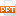# Kế toán, kiểm toán - Chapter 7: Cost - Volume - profit analysis

The Break-Even Point

The break-even point is the point in the volume of activity where

the organization’s revenues and expenses are equal.15 trang | Chia sẻ: hongha80 | Lượt xem: 483 | Lượt tải: 0
Nội dung tài liệu Kế toán, kiểm toán - Chapter 7: Cost - Volume - profit analysis, để tải tài liệu về máy bạn click vào nút DOWNLOAD ở trên
Chapter 7Cost-Volume-Profit AnalysisThe Break-Even PointThe break-even point is the point in the volume of activity where the organization’s revenues and expenses are equal.Equation ApproachSales revenue – Variable expenses – Fixed expenses = ProfitUnitsalespriceSalesvolumein units×UnitvariableexpenseSalesvolumein units×(\$500 × X)(\$300 × X)––\$80,000 = \$0(\$200X)–\$80,000 = \$0X = 400 surf boardsContribution-Margin Approach For each additional surf board sold, Curl generates \$200 in contribution margin.Consider the following information developed by the accountant at Curl, Inc.:Contribution-Margin Approach Fixed expenses Unit contribution margin =Break-even point(in units) \$80,000 \$200= 400 surf boardsContribution-Margin Approach Here is the proof!400 × \$500 = \$200,000400 × \$300 = \$120,000Contribution Margin RatioCalculate the break-even point in sales dollars rather than units by using the contribution margin ratio. Contribution margin Sales= CM Ratio Fixed expense CM RatioBreak-even point (in sales dollars)=Contribution Margin Ratio \$80,000 40%\$200,000 sales=Graphing Cost-Volume-Profit RelationshipsViewing CVP relationships in a graph gives managers a perspective that can be obtained in no other way.Consider the following information for Curl, Inc.:Cost-Volume-Profit GraphFixed expensesTotal expensesTotal salesBreak-evenpointProfit areaLoss areaTarget Net Profit We can determine the number of surfboards that Curl must sell to earn a profit of \$100,000 using the contribution margin approach. Fixed expenses + Target profit Unit contribution margin=Units sold to earnthe target profit \$80,000 + \$100,000 \$200= 900 surf boardsSee the Equation Approach example in text book (LO1)Applying CVP AnalysisSafety MarginThe difference between budgeted sales revenue and break-even sales revenue.The amount by which sales can drop before losses begin to be incurred.See example the Safety Margin example in text book (LO4)What would happen to BREAK EVEN POINT if there is a: Changes in Fixed Costs: See example in text book (LO4)Changes in Unit Contribution Margin: See example in text book (LO4) for:Unit Variable expensesSale pricesPredicting Profit Given Expected VolumeFixed expensesUnit contribution marginTarget net profitFind: {req’d sales volume}Given:Fixed expensesUnit contribution marginExpected sales volumeFind: {expected profit}Given:See the example in text book (LO4)CVP Analysis with Multiple ProductsFor a company with more than one product, sales mix is the relative combination in which a company’s products are sold.Different products have different selling prices, cost structures, and contribution margins. See the example in text book (LO5)

Các file đính kèm theo tài liệu này:

•chap007_7224_2242.ppt
Tài liệu liên quan